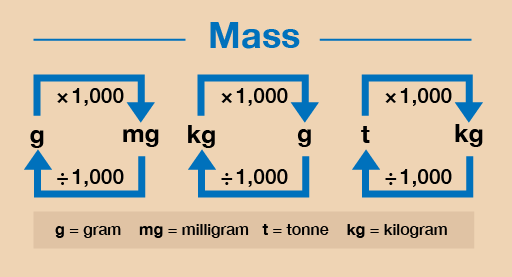Everyday maths 1 (Wales)

Start this free course now. Just create an account and sign in. Enrol and complete the course for a free statement of participation or digital badge if available.

# 3.3 Converting metric units of weight

There are occasions where you may have to convert between metric units of weight. Figure 25 shows you how to do this. In this section, we are only going to practise converting between grams (g) and kilograms (kg).

Hint: Weight is sometimes referred to as mass.Figure 25 A conversion chart for weight

## Example: Converting units of weight

1. Convert the following from kilograms into grams:
• a.4 kg = ? g
• b.6.5 kg = ? g
2. Convert the following from grams into kilograms:
• a.8 000 g = ? kg
• b.1 250 g = ? kg

### Method

1. As you can see from Figure 25, to convert from kilograms (kg) to grams (g) you need to multiply by 1 000:
• a.4 kg × 1 000 = 4 000 g
• b.6.5 kg × 1 000 = 6 500 g
2. If you want to convert from grams (g) to kilograms (kg) you need to divide by 1 000:
• a.8 000 g ÷ 1 000 = 8 kg
• b.1 250 g ÷ 1 000 = 1.25 kg

Now try the following activity.

## Activity 13: Converting metric units of weight

Calculate the following without using a calculator. You may wish to look back at Session 1 to remind you how to multiply [Tip: hold Ctrl and click a link to open it in a new tab. (Hide tip)] and divide by 1 000.

1. Convert the following to kilograms:
• a.3 000 g
• b.9 500 g
• c.750 g
• d.10 000 g
2. Convert the following to grams:
• a.4 kg
• b.1.5 kg
• c.7.6 kg
• d.2.25 kg

1. The answers are as follows:
• a.3 000 g ÷ 1 000 = 3 kg
• b.9 500 g ÷ 1 000 = 9.5 kg
• c.750 g ÷ 1 000 = 0.75 kg
• d.10 000 g ÷ 1 000 = 10 kg
2. The answers are as follows:
• a.4 kg × 1 000 = 4 000 g
• b.1.5 kg × 1 000 = 1 500 g
• c.7.6 kg × 1 000 = 7 600 g
• d.2.25 kg × 1 000 = 2 250 g#### Live in Wales? Free your ambition with a paid part-time course with the OU in Wales.

With grants of up to £4,500* to help with living costs, and tuition fee loans to cover course fees,
now's the time to take that next step.

#### Find out more

*Eligibility rules apply for financial support.